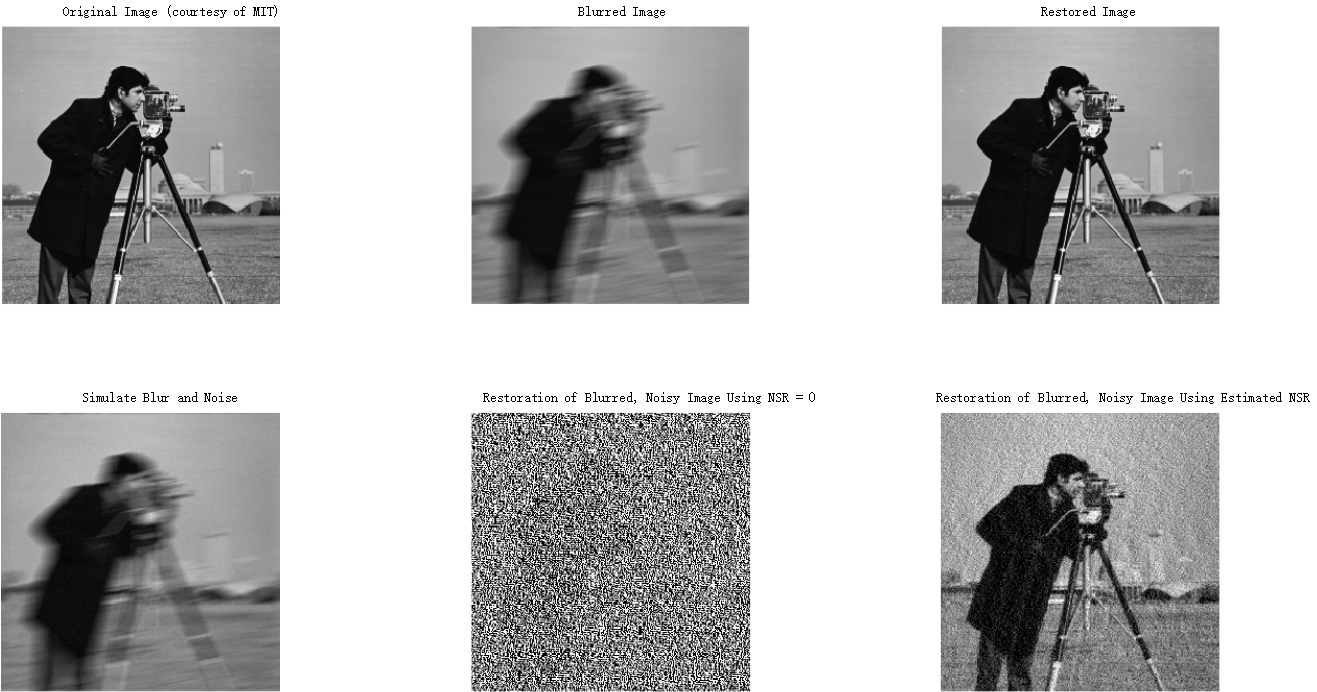# 图像去模糊（维纳滤波）

## 定义

y(t)=h(t)x(t)+n(t)

• x(t)$x(t)$是在时间t$t$刻输入的信号（未知）
• h(t)$h(t)$是一个线性时间不变系统的脉冲响应（已知）
• n(t)$n(t)$是加性噪声，与x(t)$x(t)$不相关（未知）
• y(t)$y(t)$是我们观察到的信号
我们的目标是找出这样的卷积函数g(t)$g(t)$，这样我们可以如下得到估计的x(t)$x(t)$
x^(t)=g(t)y(t)

这里x^(t)$\hat x(t)$x(t)$x(t)$的最小均方差估计。
基于这种误差度量， 滤波器可以在频率域如下描述
G(f)=H(f)S(f)|H(f)|2S(f)+N(f)=H(f)|H(f)|2+N(f)/S(f)

这里：
• G(f)$G(f)$H(f)$H(f)$g$g$h$h$在频率域f$f$的傅里叶变换。
• S(f)$S(f)$是输入信号x(t)$x(t)$的功率谱。
• N(f)$N(f)$是噪声的n(t)$n(t)$的功率谱。
• 上标$*$代表复数共轭。
滤波过程可以在频率域完成：
X^(f)=G(f)Y(f)

这里 X^(f)$\hat X(f)$x^(t)$\hat x(t)$的傅里叶变换，通过逆傅里叶变化可以得到去卷积后的结果x^(t)$\hat x(t)$

## 解释

G(f)=1H(f)|H(f)|2|H(f)|2+N(f)S(f)=1H(f)|H(f)|2|H(f)|2+1SNR(f)

## 推导

e(f)=E|X(f)X^(f)|2

e(f)=E|X(f)G(f)Y(f)|2=E|X(f)G(f)[H(f)X(f)+V(f)]|2=E|[1G(f)H(f)]X(f)G(f)V(f)|2

e(f)=[1G(f)H(f)][1G(f)H(f)]E|X(f)|2[1G(f)H(f)]G(f)E{X(f)V(f)}G(f)[1G(f)H(f)]E{V(f)X(f)}+G(f)G(f)E|V(f)|2

E{X(f)V(f)}=E{V(f)X(f)}=0

S(f)=E|X(f)|2N(f)=E|V(f)|2

e(f)=[1G(f)H(f)][1G(f)H(f)]S(f)+G(f)G(f)N(f)

d(f)dG(f)=G(f)N(f)H(f)[1G(f)H(f)]S(f)=0

## 测试

Matlab自带了示例程序，如下

%Read image
I = im2double(imread('cameraman.tif'));
figure,subplot(2,3,1),imshow(I);
title('Original Image (courtesy of MIT)');

%Simulate a motion blur
LEN = 21;
THETA = 11;
PSF = fspecial('motion', LEN, THETA);
blurred = imfilter(I, PSF, 'conv', 'circular');
subplot(2,3,2),imshow(blurred);
title('Blurred Image');

%Restore the blurred image
wnr1 = deconvwnr(blurred, PSF, 0);
subplot(2,3,3),imshow(wnr1);
title('Restored Image');

%Simulate blur and noise
noise_mean = 0;
noise_var = 0.0001;
blurred_noisy = imnoise(blurred, 'gaussian', ...
noise_mean, noise_var);
subplot(2,3,4),imshow(blurred_noisy)
title('Simulate Blur and Noise')

%Restore the blurred and noisy image:First attempt
wnr2 = deconvwnr(blurred_noisy, PSF, 0);
subplot(2,3,5);imshow(wnr2);title('Restoration of Blurred, Noisy Image Using NSR = 0')

%Restore the Blurred and Noisy Image: Second Attempt
signal_var = var(I(:));
wnr3 = deconvwnr(blurred_noisy, PSF, noise_var / signal_var);
subplot(2,3,6),imshow(wnr3)
title('Restoration of Blurred, Noisy Image Using Estimated NSR');